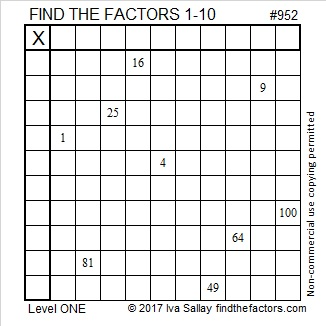# 952 and Level 1

If you’ve never solved a Find the Factors puzzle before, this one will be perfect for you to do. It only has nine clues, but that is enough to find all the factors and fill in the entire multiplication table. You will feel quite smart when you’re done, too.Print the puzzles or type the solution in this excel file: 10-factors-951-958

This is my 952nd post so I will mention a few facts about that number.

Stetson.edu informs us that 93 + 53 + 23 + 9 × 5 × 2 = 952

952 is the hypotenuse of a Pythagorean triple:
448-840-952 which is (8-15-17) times 56

952 looks interesting in some other bases:
4224 in BASE 6 because 4(6³) + 2(6²) + 2(6¹) + 4(6⁰) = 952
2C2 in BASE 19 (C is 12 Base 10) because 2(19²) + 12(19¹) +2(19⁰) = 952
SS BASE 33 (S is 28) because 28(33¹) + 28(33⁰) = 28(33 + 1) = 28(34) = 952
S0 BASE 34 because 28(34¹) + 0(34⁰) = 28(34) = 952

• 952 is a composite number.
• Prime factorization: 952 = 2 × 2 × 2 × 7 × 17, which can be written 952 = 2³ × 7 × 17
• The exponents in the prime factorization are 3, 1, and 1. Adding one to each and multiplying we get (3 + 1)(1 + 1)(1 + 1) = 4 × 2 × 2 = 16. Therefore 952 has exactly 16 factors.
• Factors of 952: 1, 2, 4, 7, 8, 14, 17, 28, 34, 56, 68, 119, 136, 238, 476, 952
• Factor pairs: 952 = 1 × 952, 2 × 476, 4 × 238, 7 × 136, 8 × 119, 14 × 68, 17 × 56, or 28 × 34
• Taking the factor pair with the largest square number factor, we get √952 = (√4)(√238) = 2√238 ≈ 29.854497